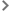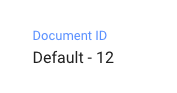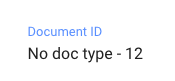# Getting started with formulas for calculated properties

Calculated properties are custom properties whose values are calculated from a formula. The formula can include the values of other properties, thereby creating dependencies between your properties.

Notes:
– This feature is currently available as a private beta and will be available as a public beta at a later date.

This article explains how to implement simple use cases with code examples you can copy.Before you startCreate a unique document sequence IDWhat is the sequence ID?Create a sequence ID for all documents in a document classCreate a document sequence ID with a fixed prefixCreate a document sequence ID with a prefix issued from a String propertyCreate a document sequence ID with a prefix issued from a Category propertyCreate a document sequence ID prefixed with the document creation yearUse a date with a specific formatUse a Date system property with the format YYYY/MM/DDUse a Date custom property with the format MM/DD/YYYYCalculate a "next review" dateCalculate a "next review" date as a fixed period after the "Application date"Calculate a "next review" date as "Review period" (number of months) after the "Application date"Calculate a duration between two datesUse simple math calculations

## Before you start

You can use existing properties in your formulas. To do this, when you create or edit your calculated property, select the required property in the left panel of the Formula pop-up. A block of text with a unique identifier is inserted into your formula, like this:

`doc.fields['#{field:S00kJlFArg9Qmjpw6X}']`

This block of text is called an entity reference. If you copy the examples provided in this article, make sure you don't select the entity references. When you insert your own properties into your formulas, unique entity references are generated.

## Create a unique document sequence ID

### What is the sequence ID?

In AODocs, each version of a document is given a set of four numbers, composed of the following:

• Library sequence ID
The first library created in your domain has the library sequence ID `1`, the second has `2`, etc.
• Document class sequence ID
The first document class in a library has the document class sequence ID `1`, the second has`2`, etc.
• Document sequence ID
The first document created in a document class has the document sequence ID `1`, the second has `2`, etc.
• Version sequence ID
The first version of a document has the version sequence ID `1`, the second version has `2`, etc.

For example, the full sequence ID `34, 3, 897, 2` corresponds to the 2nd version of the 897th document of the 3rd class of the 34th library in the domain.

Important:
– Full sequence IDs are unique per document version within a domain.
– Sequence IDs are generated sequentially. However, if an error occurs, a given number may be skipped.

Sequence IDs are useful because they allow you to generate unique IDs that you can use to reference your documents. For example, you can save the document sequence ID in a calculated property. This gives you automatically generated and easily accessible document references.

You can use library, document class and document sequence IDs in formulas for calculated properties.

### Create a sequence ID for all documents in a document class

Note: This generates a unique ID per document within a document class.

1. Create a new String type calculated property. Name it "Document ID", for example.

2. Write the following formula:

`'#{seqId.doc}'`

If you want a document sequence ID with a specific number of digits, replace your formula by:

`'#{seqId.doc}'.padStart(5,'0')`

where `5` is the number of digits you want.

### Create a document sequence ID with a fixed prefix

Use case: Give the prefix "SOP" to all the document sequence IDs of your "Standard Operating Procedure" document class.

1. Create a new String type calculated property. Name it "Document ID", for example.

2. Write the following formula:

`'SOP'+' - '+'#{seqId.doc}'`

If you want the number part of the document sequence ID to have a specific number of digits, replace `'#{seqId.doc}'` by `'#{seqId.doc}'.padStart(5,'0')`

where `5` is the number of digits you want.

### Create a document sequence ID with a prefix issued from a String property

Use case: Make all the document sequence IDs of your "Project" document class start with the value of the "Project name" property (type String).

Example of the final formula:

``` doc.fields['#{field:S00kJlFArg9Qmjpw6X}']+' - '+'#{seqId.doc}' ```

1. Create a new String type calculated property. Name it "Document ID", for example.

2. Press the name of the String type property (in our example, "Project name"), listed in the left panel of the Formula pop-up, under String. An entity reference like this is inserted into the body of the formula:

`doc.fields['#{field:S00kJlFArg9Qmjpw6X}']`

3. Write the rest of the formula:

`+' - '+'#{seqId.doc}'`

If you want the number part of the ID to have a specific number of digits, replace `'#{seqId.doc}'` by `'#{seqId.doc}'.padStart(5,'0')`

where `5` is the number of digits you want.

Important: If there is no value in the String type property ("Project name" in our example), its entity reference is replaced with `null`. You can write this formula to replace the entity reference by a value of your choice ("Default", in our example):
`(doc.fields['#{field:S00kJlFArg9Qmjpw6X}'] || 'Default') +' - '+'#{seqId.doc}'`
For example:### Create a document sequence ID with a prefix issued from a Category property

Use case: Make all the document sequence IDs of your "Document" document class start with the value of the "Document type" property (type Category).

Note: This formula only works with mono-value, single-level properties. Learn more: What are categories?

Example of the final formula:

`doc.fields['#{field:Rxe3BdXxKMdXmJGKPR}'].name +' - '+'#{seqId.doc}'`

1. Create a new String type calculated property. Name it "Document ID", for example.

2. Press the name of the Category type property (in our example, "Document type"), listed in the left panel of the Formula pop-up, under Category. An entity reference like this is inserted into the body of the formula:

`doc.fields['#{field:Rxe3BdXxKMdXmJGKPR}']`

3. Write the rest of the formula:

`.name +' - '+'#{seqId.doc}'`

If you want all the sequence IDs of your "Document" document class to start with the short name of the value of the "Document type" property category, replace `.name` by `.shortName`. Final result:

``` doc.fields['#{field:Rxe3BdXxKMdXmJGKPR}'].shortName +' - '+'#{seqId.doc}' ```

If you want the number part of the document sequence ID to have a specific number of digits, replace `'#{seqId.doc}'` by `'#{seqId.doc}'.padStart(5,'0')`

where `5` is the number of digits you want.

Important: If there is no value in the Category property, an error occurs when the formula is calculated, preventing the document from being saved. Use this formula to replace the category's entity reference by a value of your choice ("No doc type" in our example):
``` (doc.fields['#{field:Rxe3BdXxKMdXmJGKPR}'] && doc.fields['#{field:Rxe3BdXxKMdXmJGKPR}'].name|| 'No doc type')+" - "+'#{seqId.doc}' ```
For example:### Create a document sequence ID prefixed with the document creation year

1. Create a new String type calculated property. Name it "Document ID", for example.

2. Write the following formula:

``` new Date(doc.creationDate).getFullYear()+' - '+'#{seqId.doc}' ```

If you want the number part of the ID to have a specific number of digits, replace `'#{seqId.doc}'` by `'#{seqId.doc}'.padStart(5,'0')`

where `5` is the number of digits you want.

## Use a date with a specific format

### Use a Date system property with the format YYYY/MM/DD

Use case: Use the document creation date in a calculated property with the following format: YYYY/MM/DD

Final formula:

``` (() => { const d=new Date(doc.creationDate) const yyyy=d.getFullYear() const mm=`\${d.getMonth()+1}`.padStart(2,'0') const dd=`\${d.getDate()}`.padStart(2,'0') return `\${yyyy}/\${mm}/\${dd}` })() ```

### Use a Date custom property with the format MM/DD/YYYY

Use case: Use the value of the "Publication date" property in your calculated property with the following format: MM/DD/YYYY

Example of the final formula:

``` (() => { const d=new Date(doc.fields['#{field:Rxe3BdXxKMdXmJGKPR}']) const yyyy=d.getFullYear() const mm=`\${d.getMonth()+1}`.padStart(2,'0') const dd=`\${d.getDate()}`.padStart(2,'0') return `\${mm}/\${dd}/\${yyyy}` })() ```

1. Create a new String type calculated property. Name it "Formatted publication date", for example.

2. Write the following formula:

``` (() => { const d=new Date( ```

3. Press the name of the Date type property (in our example, "Publication date"), listed in the left panel of the Formula pop-up under Date.

An entity reference like this is inserted in the body of the formula:

`doc.fields['#{field:Rxe3BdXxKMdXmJGKPR}']`

4. Write the rest of the formula:

``` ) const yyyy=d.getFullYear() const mm=`\${d.getMonth()+1}`.padStart(2,'0') const dd=`\${d.getDate()}`.padStart(2,'0') return `\${mm}/\${dd}/\${yyyy}` })()```

## Calculate a "next review" date

This formula lets you to generate a date in a calculated property from another date property to which a given number of months is added.

### Calculate a "next review" date as a fixed period after the "Application date"

Use case: Calculate a "next review" date, 12 months after the "Application date".

Example of the final formula:

``` (() => { var date = new Date(doc.fields['#{field:RgBi2MO0Epea1Xkbp2}']); date.setMonth(date.getMonth()+12); return date; })() ```

1. Create a new Date type calculated property. Name it "Next review date", for example.

2. Write the following formula:

``` (() => { var date = new Date( ```

3. Press the name of the Date type property (in our example, "Application date"), listed in the left panel of the Formula pop-up, under Date. An entity reference like this is inserted in the body of the formula:

``` doc.fields['#{field:RgBi2MO0Epea1Xkbp2}'] ```

4. Write the rest of the formula:

``` ); date.setMonth(date.getMonth()+12); return date; })() ```

### Calculate a "next review" date as "Review period" (number of months) after the "Application date"

Use case: Generate a "next review" date calculated from the number of months in the "Review period (in months)" property after the "Application date".

Example of the final formula:

``` (() => { var date = new Date(doc.fields['#{field:RgBi2MO0Epea1Xkbp2}']); date.setMonth(date.getMonth()+doc.fields['#{field:RfFxvD8uR1UWFvHDnm}']); return date; })() ```

1. Create a new Date type calculated property. Name it "Next review date", for example.

2. Write the following formula:

``` (() => { var date = new Date( ```

3. Press the name of the Date type property (in our example, "Application date"), listed in the left panel of the Formula pop-up, under Date. An entity reference like this is inserted into the body of the formula:

```doc.fields['#{field:Ry1zrxa8eRCWHn9RP7}'] ```

4. Add this to the formula:

``` ); date.setMonth(date.getMonth()+ ```

5. Press the name of the Integer type property (in our example, "Review period (in months)"), listed in the left panel of the Formula pop-up, under Integer. An entity reference like this is inserted into the body of the formula:

```doc.fields['#{field:RfFxvD8uR1UWFvHDnm}'] ```

6. Write the rest of the formula:

``` ); return date; })() ```

## Calculate a duration between two dates

Use case: Calculate how many days between the "Start Date" and "End Date", defined in your document as Date type properties.

Note: The value calculated can be negative.

Example of the final formula:

``` (() => { var startDate = new Date(doc.fields['#{field:Ry1zrxa8eRCWHn9RP7}']); var returnDate = new Date(doc.fields['#{field:RxMLGItwuaVmm5A8el}']); return (returnDate - startDate) / (1000 * 3600 * 24); })() ```

1. Create a new Integer type calculated property. Name it "Duration (in days)", for example.

2. In the formula, write the following:

``` (() => { var startDate = new Date( ```

3. Press the name of the Date type property (in our example "Start Date"), listed in the left panel of the Formula pop-up, under Date. An entity reference like this is inserted into the body of the formula:

```doc.fields['#{field:Ry1zrxa8eRCWHn9RP7}'] ```

4. Add this to the formula:

``` ); var returnDate = new Date( ```

5. Select the Date type property (in our example, "End Date"), listed in the left panel of the Formula pop-up, under Date. An entity reference like this is inserted in the body of the formula:

```doc.fields['#{field:RxMLGItwuaVmm5A8el}'] ```

6. Write the following at the end of the formula:

``` ); return (returnDate - startDate) / (1000 * 3600 * 24); })() ```

## Use simple math calculations

Use cases:

• Calculate the total price of a commercial proposal, for example:
• number of days × daily rate
• number of pieces × price per piece
• Calculate the VAT with a fixed tax percentage
• Calculate the VAT with a tax percentage written in another property

It's easy to write a math calculation for the formula of a calculated properties. You can use the standard operators: +, -, *, /

To use Number type properties (Integer or Decimal), press the property name in the left panel of the Formula pop-up, under Integer or Decimal. When you do this, an entity reference like this is inserted in the body of the formula:

`doc.fields['#{field:Ry1zrxa8eRCWHn9RP7}']`

The formula of a sum between two properties looks like this:

``` doc.fields['#{field:Ry1zrxa8eRCWHn9RP7}'] + doc.fields['#{field:RxMLGItwuaVmm5A8el}'] ```

If you need to use fixed numbers, simply write them in the formula:

``` 1.2 * (doc.fields['#{field:Ry1zrxa8eRCWHn9RP7}'] + doc.fields['#{field:RxMLGItwuaVmm5A8el}']) ```

Have more questions? Submit a request

1 comment
• Hello, I have one questions regarding the sequence ID :
I created one document class for correspondence, created some documents inside to test and ajust the parameters, then deleted them.
Then I implemented one calculated metadata to generate a sequence number. When I started to create new documents, the chrono started at 012, which i guess is because it's taking into account the old documents, even though I permanently deleted them.
1- Is there a way to make the sequence start at 001 ?
2- Moreover, is there a way to start the chrono at a chosen number ? (for example if documents with a sequence number were already existing prior to the creation of the aodocs site) ?